Question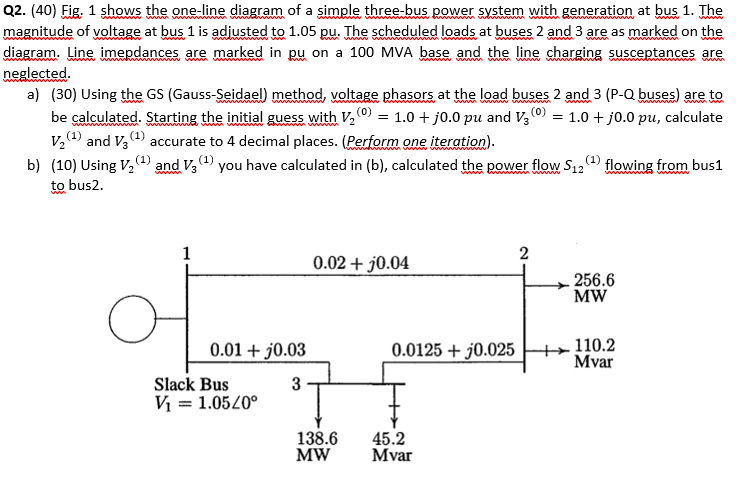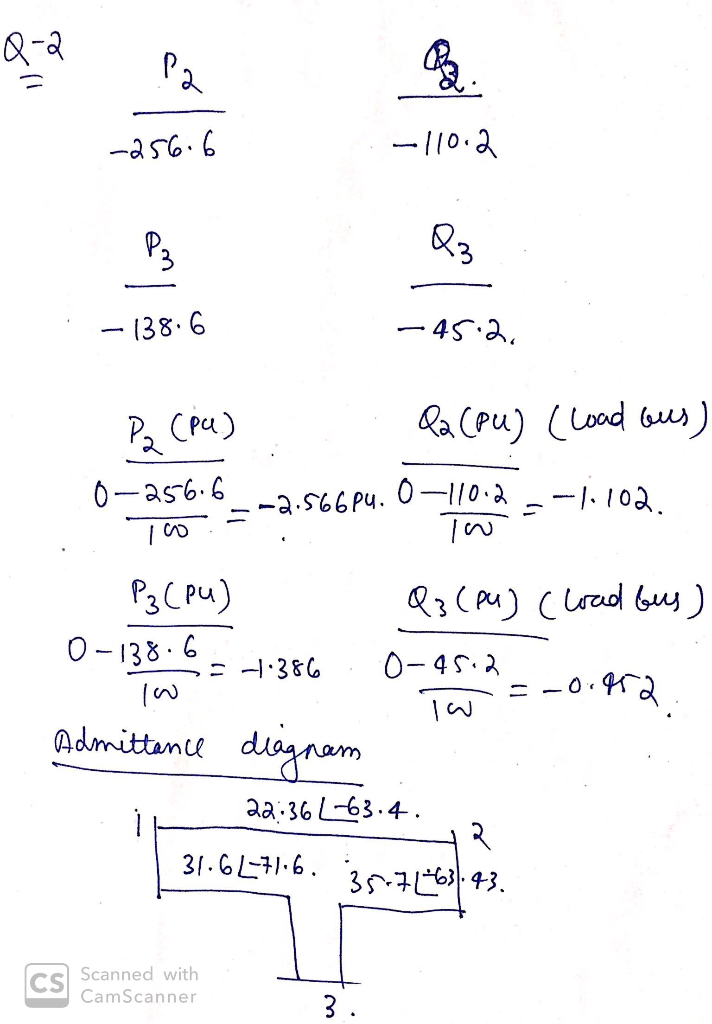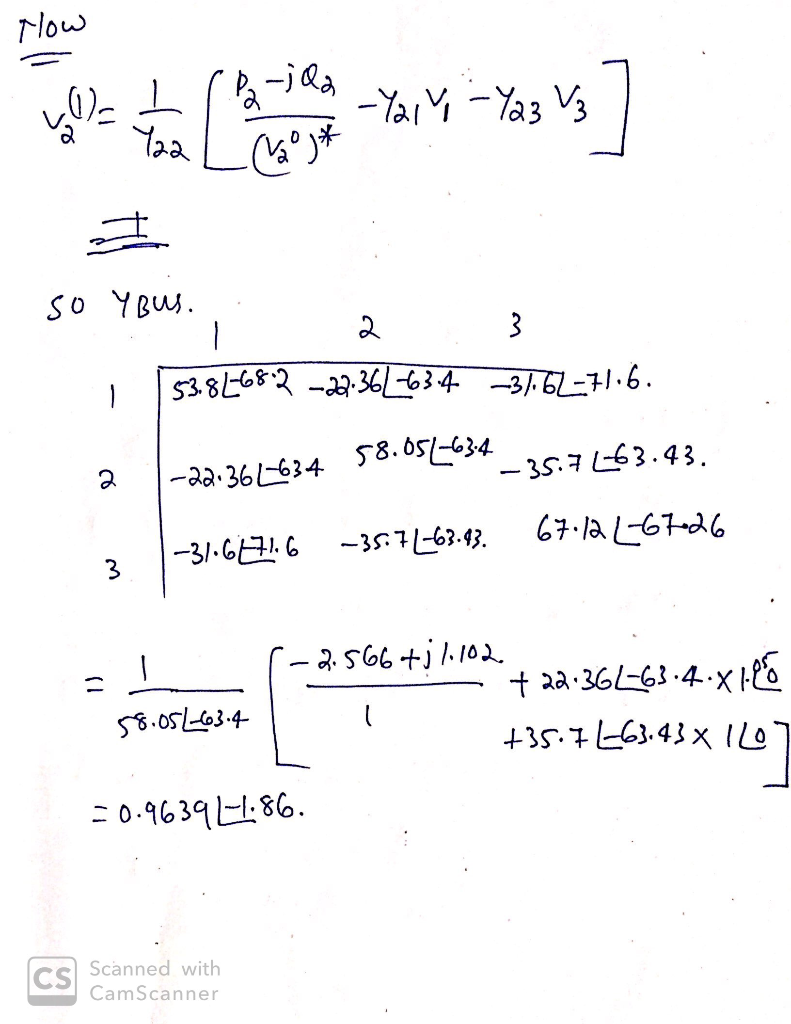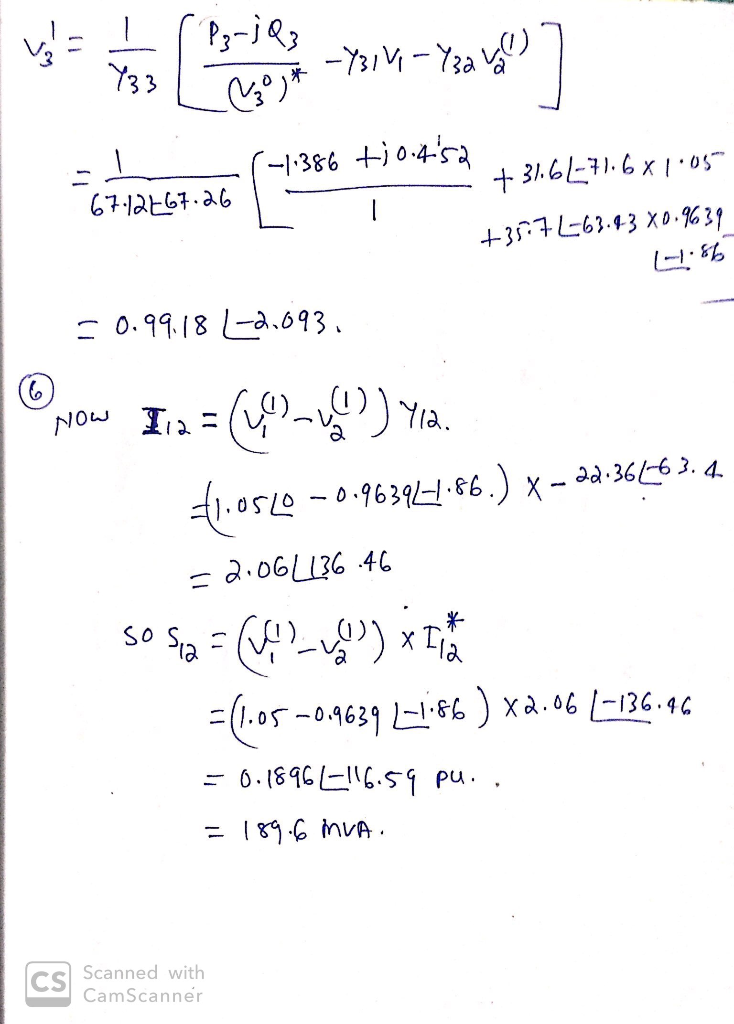#### Earn Coins

Coins can be redeemed for fabulous gifts.

Similar Homework Help Questions
• ### Q2. i) The one-line diagram of simple three-bus power system with generation at bus 1 is shown in figure Q2. 0.02 + 30.04 2 256.6 MW 0.0125 + 30.025 +110.2 Mvar 0.01 + 30.03 Slack Bus 3 Vi = 1.0520° 138.6 MW 45.2 Mvar Figure Q2 The magnitude of voltage at

Q2. i) The one-line diagram of simple three-bus power system with generation at bus 1 is shown in figure Q2. 0.02 + 30.04 2 256.6 MW 0.0125 + 30.025 +110.2 Mvar 0.01 + 30.03 Slack Bus 3 Vi = 1.0520° 138.6 MW 45.2 Mvar Figure Q2 The magnitude of voltage at bus 1 is adjusted to 1.05 per unit. The scheduled loads at buses 2 and 3 are as marked on the diagram. Line impedances are marked in per unit...

• ### 3. Figure 3, shows the one-line diagram of a simple three-bus power system with generation at buses 1 and 3. The voltag...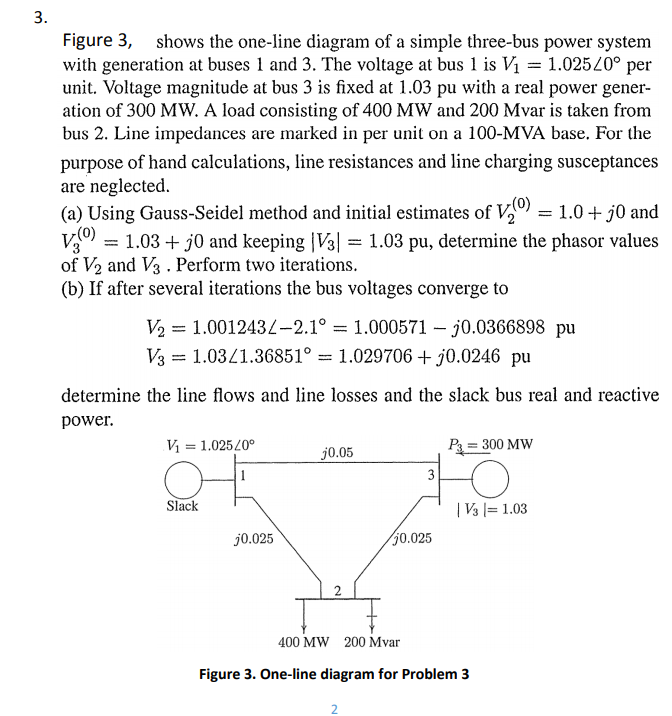Figure 3, shows the one-line diagram of a simple three-bus power system with generation at buses 1 and 3 . The voltage at bus 1 is $$V_{1}=1.025 \angle 0^{\circ}$$ per unit. Voltage magnitude at bus 3 is fixed at $$1.03$$ pu with a real power generation of $$300 \mathrm{MW}$$. A load consisting of $$400 \mathrm{MW}$$ and $$200 \mathrm{Mvar}$$ is taken from bus 2. Line impedances are marked in per unit on a 100-MVA base. For the purpose of hand calculations,...

• ### Consider the single line diagram of a 3-bus power system shown in Figure 2. Slack bus...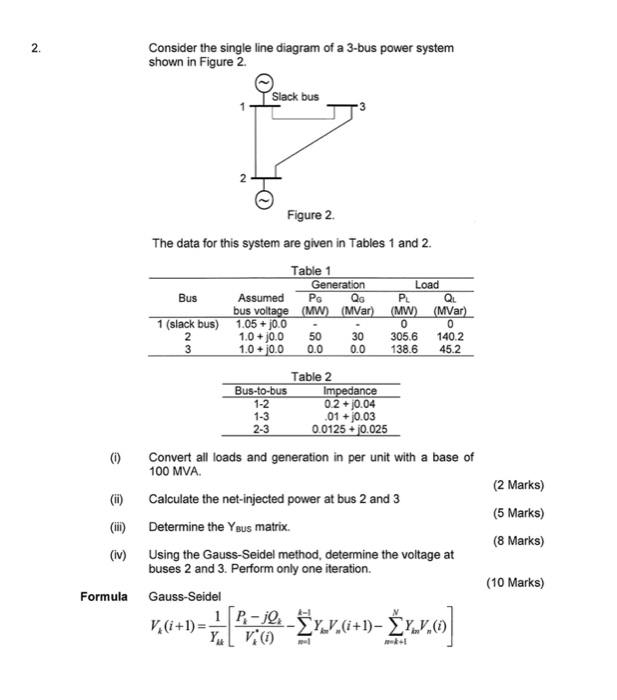Consider the single line diagram of a 3-bus power system shown in Figure 2. Slack bus 3 Figure 2. The data for this system are given in Tables 1 and 2. Bus Table 1 Generation Load Assumed PG QGPLQL bus voltage (MW) (MVar) (MW) (MVar) 1.05 +10.0 - - 1.0 + 0.0 50 30 305.6 140.2 1.0 +0.0 0.0 0.0 138.6 45.2 slack bus) Table 2 Bus-to-bus Impedance 0.2 + j0.04 .01 +0.03 2.3 0.0125 + j0.025 (0) Convert all...

• ### Figure 1 shows the one line diagram of a simple power system. Generators are connected at...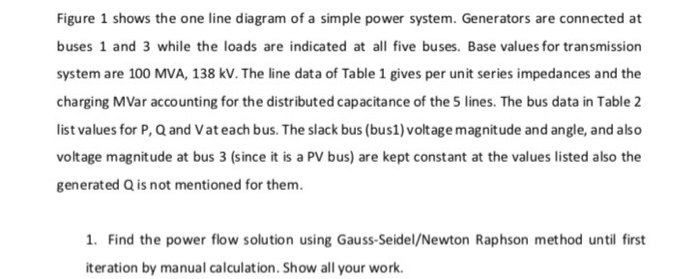Figure 1 shows the one line diagram of a simple power system. Generators are connected at buses 1 and 3 while the loads are indicated at all five buses. Base values for transmission system are 100 MVA, 138 kV. The line data of Table 1 gives per unit series impedances and the charging MVar accounting for the distributed capacitance of the 5 lines. The bus data in Table 2 list values for P, Q and Vat each bus. The slack...

• ### The one line diagram of a three-phase power system is shown in Fig.8. Impedances are marked...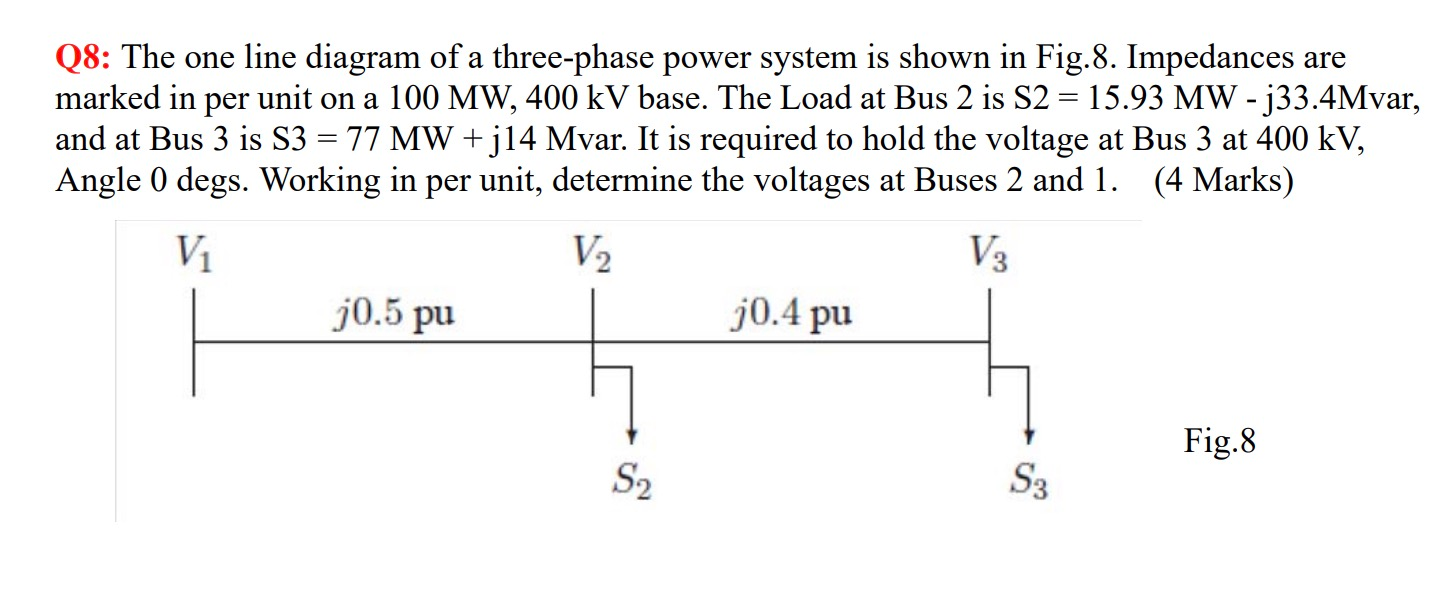The one line diagram of a three-phase power system is shown in Fig.8. Impedances are marked in per unit on a 100 MW, 400 kV base. The Load at Bus 2 is S2 = 15.93 MW - j33.4Mvar, and at Bus 3 is S3 = 77 MW + j14 Mvar. It is required to hold the voltage at Bus 3 at 400 kV, Angle 0 degs. Working in per unit, determine the voltages at Buses 2 and 1. Q8: The...

• ### Question 1: A single line diagram of a three-bus power system is shown in Fig 1....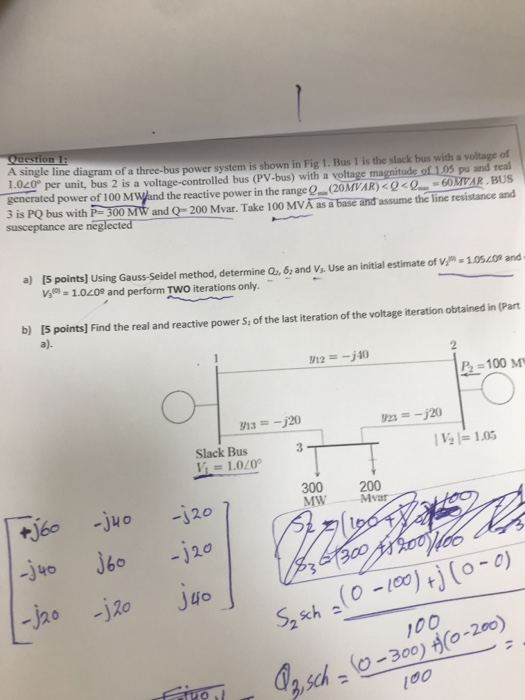Question 1: A single line diagram of a three-bus power system is shown in Fig 1. Bus 1 is the slack bus with a voltage of 1.020 per unit, bus 2 is a voltage-controlled bus (PV-bus) with a voltage magnitude of 1.05 pu and real generated power of 1 00 MWand the reactive power in the range Q.(20MVAR) < Q<Q-60M¥AR .BUS 3 is PQ bus with P 300 MW and Q= 200 Mvar. Take 100 MVÅ susceptance are neglected as...

• ### 3- The figure given below shows the one line diagram of a simple power system. Line impedançe is given in per unit...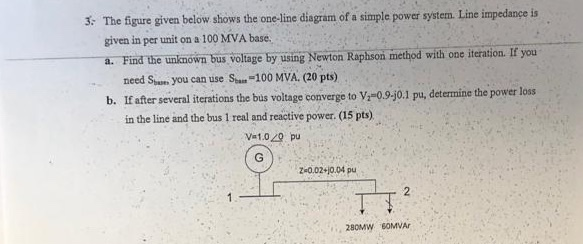3- The figure given below shows the one line diagram of a simple power system. Line impedançe is given in per unit on a 100 MVA base. a. Find the unknown bus voltage by using Newton Raphson method with one iteration. IE you need Ssum you can use San-100 MVA. (20 pts) b. If after several iterations the bus voltage converge to V09-j0.1 pu, determine the power loss in the line and the bus 1 real and reactive power. (15...

• ### This is 5 bus ssystem white full answer I will like your a Figure 1 shows the one line diagram of...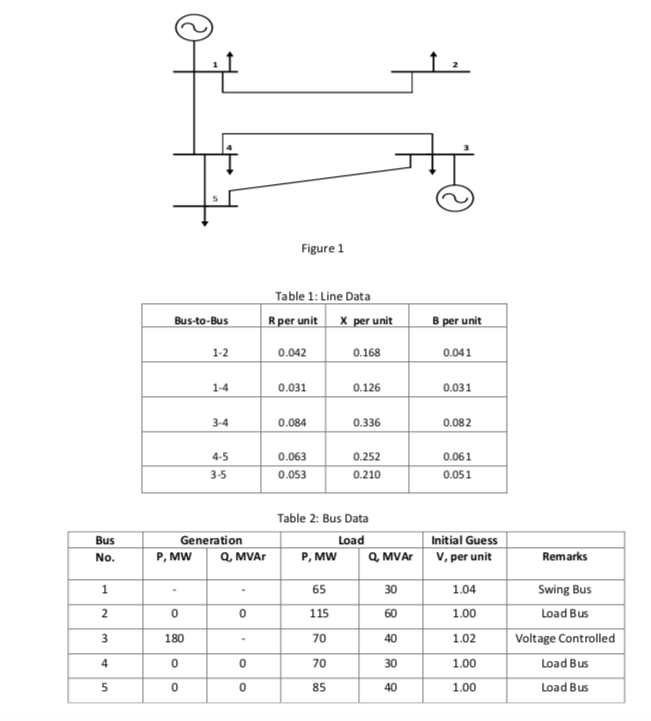this is 5 bus ssystem white full answer I will like your a Figure 1 shows the one line diagram of a simple power system. Generators are connected at buses 1 and 3 while the loads are indicated at all five buses. Base values for transmission system are 100 MVA, 138 kV. The line data of Table 1 gives per unit series impedances and the charging MVar accounting for the distributed capacitance of the 5 lines. The bus data in...

• ### Problem #1 Part I (50 points) Consider the following one-line diagram of a three-phase power system. Assume that the system has the following base quantities: S3 100 MVA, and VbaselL 38 kV at the...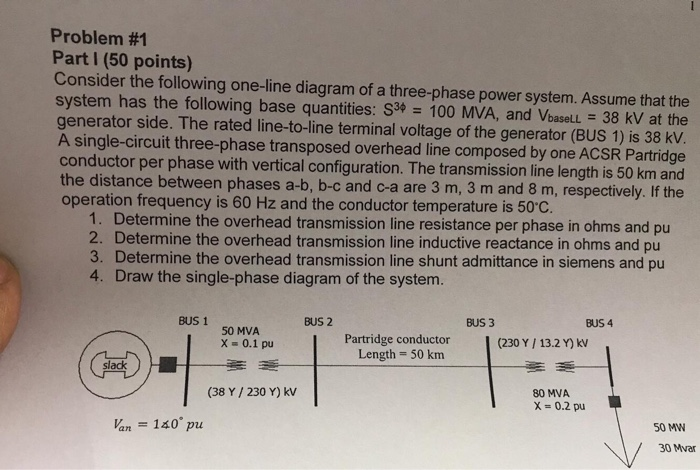Problem #1 Part I (50 points) Consider the following one-line diagram of a three-phase power system. Assume that the system has the following base quantities: S3 100 MVA, and VbaselL 38 kV at the generator side. The rated line-to-line terminal voltage of the generator (BUS 1) is 38 kV. A single-circuit three-phase transposed overhead line composed by one ACSR Partridge conductor per phase with vertical configuration. The transmission line length is 50 km and the distance between phases a-b, b-c...

• ### (POWER SYSTEM ANALYSIS) Load + Load Figure 1. One line diagram of a three bus power...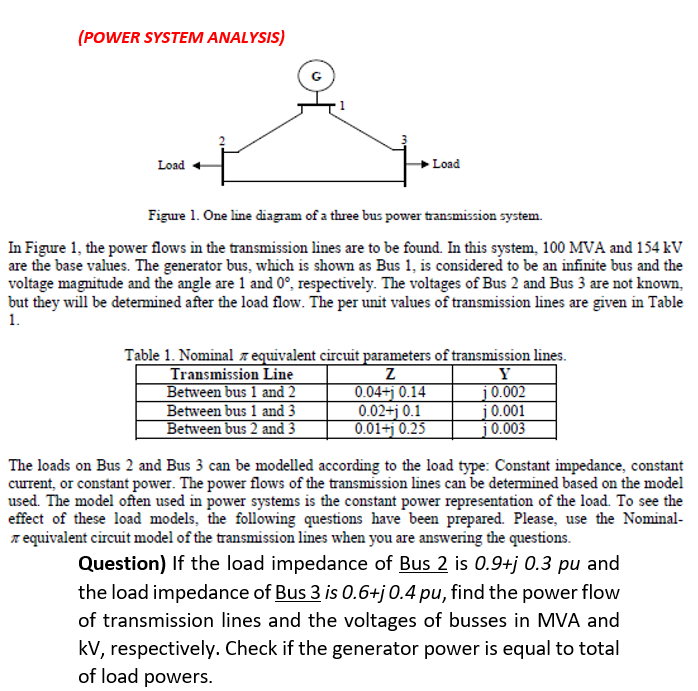(POWER SYSTEM ANALYSIS) Load + Load Figure 1. One line diagram of a three bus power transmission system. In Figure 1, the power flows in the transmission lines are to be found. In this system, 100 MVA and 154 kV are the base values. The generator bus, which is shown as Bus 1, is considered to be an infinite bus and the voltage magnitude and the angle are 1 and 0°, respectively. The voltages of Bus 2 and Bus 3...